# Quiz 6: Forecasting

a) The constant growth model with annual compounding can be described as follows: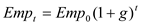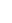…… (1) Where is employment level after time period t, is employment level in the base period and g is the annual growth rate. For the occupation of B.C we have: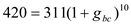Taking logs on both the sides,Take antilog on both the sides,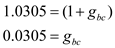Substituting this value in Equation (1)Hence annually compounded value for the occupation B.C for the year 2018 is 567. Similarly performing the same procedure for other occupations we get the following table for the annually compounded values: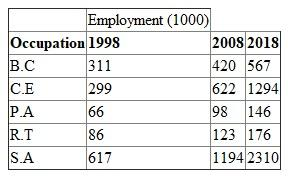The constant growth model with continuous compounding is based on the following: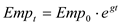…… (2) Where is employment level after time period t, is employment level in the base period and g is the annual growth rate. For the occupation B.C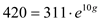Take log on both the side,Substituting in Equation (2)The continuously compounded value for occupation B.C is 567. Repeating it for other occupations we get the following results:b) The results from the two different methods i.e. constant growth model with annual compounding and continuous compounding are similar.